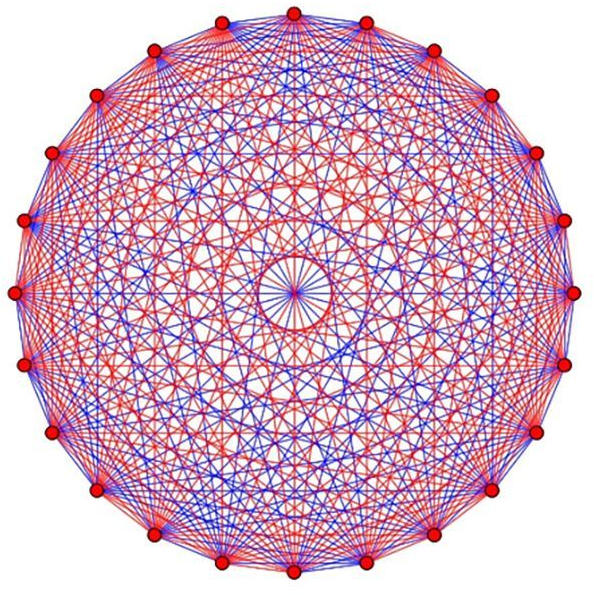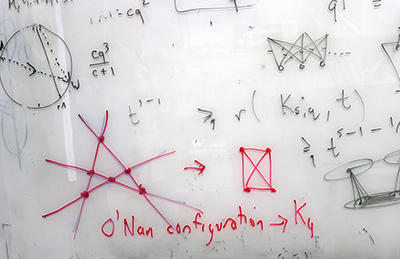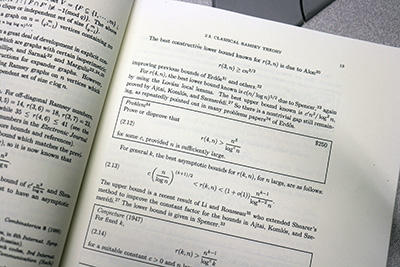# Perplexing the World for Decades – Mathematicians Unlock the Secret to Ramsey NumbersUC San Diego mathematicians Jacques Verstraete and Sam Mattheus solved a long-standing Ramsey number problem, r(4,t), utilizing pseudorandom graphs from finite geometry. Their breakthrough provides a near cubic function estimation for such Ramsey numbers.

### UC San Diego mathematicians unlock the secret to Ramsey numbers.

We’ve all been there: staring at a math test with a problem that seems impossible to solve. What if finding the solution to a problem took almost a century? For mathematicians who dabble in Ramsey theory, this is very much the case. In fact, little progress has been made in solving Ramsey problems since the 1930s.

Now, University of California San Diego researchers Jacques Verstraete and Sam Mattheus have found the answer to r(4,t), a longstanding Ramsey problem that has perplexed the math world for decades.Ramsey problems, such as r(4,5) are simple to state, but as shown in this graph, the possible solutions are nearly endless, making them very difficult to solve. Credit: Jacques Verstraete

#### What was Ramsey’s problem, anyway?

In mathematical parlance, a graph is a series of points and the lines in between those points. Ramsey theory suggests that if the graph is large enough, you’re guaranteed to find some kind of order within it — either a set of points with no lines between them or a set of points with all possible lines between them (these sets are called “cliques”). This is written as r(s,t) where s are the points with lines and t are the points without lines.

To those of us who don’t deal in graph theory, the most well-known Ramsey problem, r(3,3), is sometimes called “the theorem on friends and strangers” and is explained by way of a party: in a group of six people, you will find at least three people who all know each other or three people who all don’t know each other. The answer to r(3,3) is six.It took a pseudorandom graph from finite geometry, called an O’Nan configuration, to solve a longstanding Ramsey problem. Credit: UC San Diego

“It’s a fact of nature, an absolute truth,” Verstraete states. “It doesn’t matter what the situation is or which six people you pick — you will find three people who all know each other or three people who all don’t know each other. You may be able to find more, but you are guaranteed that there will be at least three in one clique or the other.”

What happened after mathematicians found that r(3,3) = 6? Naturally, they wanted to know r(4,4), r(5,5), and r(4,t) where the number of points that are not connected is variable. The solution to r(4,4) is 18 and is proved using a theorem created by Paul Erdös and George Szekeres in the 1930s.

Currently, r(5,5) is still unknown.

#### A good problem fights back

Why is something so simple to state so hard to solve? It turns out to be more complicated than it appears. Let’s say you knew the solution to r(5,5) was somewhere between 40-50. If you started with 45 points, there would be more than 10234 graphs to consider!

“Because these numbers are so notoriously difficult to find, mathematicians look for estimations,” Verstraete explained. “This is what Sam and I have achieved in our recent work. How do we find not the exact answer, but the best estimates for what these Ramsey numbers might be?”

Math students learn about Ramsey problems early on, so r(4,t) has been on Verstraete’s radar for most of his professional career. In fact, he first saw the problem in print in Erdös on Graphs: His Legacy of Unsolved Problems, written by two UC San Diego professors, Fan Chung and the late Ron Graham. The problem is a conjecture from Erdös, who offered \$250 to the first person who could solve it.

“Many people have thought about r(4,t) — it’s been an open problem for over 90 years,” Verstraete said. “But it wasn’t something that was at the forefront of my research. Everybody knows it’s hard and everyone’s tried to figure it out, so unless you have a new idea, you’re not likely to get anywhere.”

Then about four years ago, Verstraete was working on a different Ramsey problem with a mathematician at the University of Illinois-Chicago, Dhruv Mubayi. Together they discovered that pseudorandom graphs could advance the current knowledge on these old problems.The problem r(4,t) is a conjecture from Erdös, who offered \$250 to the first person who could solve it. Credit: UC San Diego

In 1937, Erdös discovered that using random graphs could give good lower bounds on Ramsey problems. What Verstraete and Mubayi discovered was that sampling from pseudorandom graphs frequently gives better bounds on Ramsey numbers than random graphs. These bounds — upper and lower limits on the possible answer — tightened the range of estimations they could make. In other words, they were getting closer to the truth.

In 2019, to the delight of the math world, Verstraete and Mubayi used pseudorandom graphs to solve r(3,t). However, Verstraete struggled to build a pseudorandom graph that could help solve r(4,t).

He began pulling in different areas of math outside of combinatorics, including finite geometry, algebra, and probability. Eventually, he joined forces with Mattheus, a postdoctoral scholar in his group whose background was in finite geometry.

“It turned out that the pseudorandom graph we needed could be found in finite geometry,” Verstraete stated. “Sam was the perfect person to come along and help build what we needed.”

Once they had the pseudorandom graph in place, they still had to puzzle out several pieces of math. It took almost a year, but eventually, they realized they had a solution: r(4,t) is close to a cubic function of t. If you want a party where there will always be four people who all know each other or t people who all don’t know each other, you will need roughly t3 people present. There is a small asterisk (actually an o) because, remember, this is an estimate, not an exact answer. But t3 is very close to the exact answer.

The findings are currently under review with the Annals of Mathematics. A preprint can be viewed on arXiv.

“It really did take us years to solve,” Verstraete stated. “And there were many times where we were stuck and wondered if we’d be able to solve it at all. But one should never give up, no matter how long it takes.”

Verstraete emphasizes the importance of perseverance — something he reminds his students of often. “If you find that the problem is hard and you’re stuck, that means it’s a good problem. Fan Chung said a good problem fights back. You can’t expect it just to reveal itself.”

Verstraete knows such dogged determination is well-rewarded: “I got a call from Fan saying she owes me \$250.”

Reference: “The asymptotics of r(4,t)” by Sam Mattheus and Jacques Verstraete, 23 October 2023, arXiv.
DOI: 10.48550/arXiv.2306.04007

#### 8 Commentson "Perplexing the World for Decades – Mathematicians Unlock the Secret to Ramsey Numbers"

1.John Bayer | November 8, 2023 at 6:14 am | Reply

Does all this difficult mathematics have any practical applications?

2.Obrian Suro.One | November 9, 2023 at 3:14 pm | Reply

r(3,3) = 6
r(4,4) = 18
r(5,5) = 63

3.Obrian Suro.One | November 9, 2023 at 3:40 pm | Reply

If you add the above numbers [6,18,63] you get 87
Were you to add a 900 to r(5,5) your numbers would turn out as [6,18,963]

This adds up to 987. This is a pattern and you can calculate it.

Good luck!

4.Obrian Suro.One | November 9, 2023 at 3:45 pm | Reply

1863

5.Obrian Suro.One | November 9, 2023 at 3:48 pm | Reply

r(3,3) = 6
r(4,4) = 18
r(5,5) = 63
r(6,6) = 186
r(7,7) = 567
r(8,8) = 1764
r(9,9) = 5547

r(n,n)=3^n −6

6.Obrian Suro.One | November 9, 2023 at 3:50 pm | Reply

r(10,10) = 59043
r(11,11) = 177141
r(12,12) = 531435
r(13,13) = 1594317
r(14,14) = 4782963
r(15,15) = 14348901

7.Drmrforman | November 9, 2023 at 7:20 pm | Reply

Could we rename this the Kevin Bacon problem?

8.Franki | November 9, 2023 at 9:38 pm | Reply

r(n,n)=3^n-6
r(3,3)=3^3-6=27-6=21
r(4,4)=3^4-6=81-6=75
.
.
.